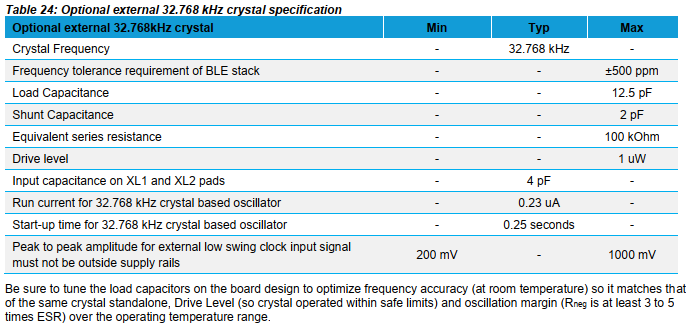# How to choose the right crystal and capacitors for your module design?

Some modules can be operated with or require adding an external crystal. To do so a suitable crystal needs to be chosen in accordance with load capacitors.

The crystal specification is usually given in the module's data sheet. See below screen shot as an example for the BL654 module.Most important parameters are the frequency, its tolerance and the load capacitance. Make sure to select a crystal matching these parameters.

As mentioned above load capacitors needs to be added to allow the module's oscillator circuit work properly and precise. So, how do you know which capacitors to chose? The load capacitance is given in the crystal data sheet and the two capacitors C1 and C2 need to match this based on the below formular:

CL = (C1 * C2) / (C1 + C2) + Cstray

Cstray represents additional (parasitic) capacitance coming from the PCB traces plus the input capacitance contributed by the crystal oscillator pads of the module. The above table gives 4pF as input capacitance and we might want to guestimate 1-2pF as capacitance from the signal traces (this depends on the particular layout...).

A commonly stated rule of thumb is to start with two capacitors of twice the load capacitance of the crystal. Assuming a 12.5pF crystal this would be 25pF for C1, C2 and we get:

CL = (25pF * 25pF) / (25pF + 25pF) + 5pF = 17.5pF

17.5pF would be significantly higher than the required 12.5pF. Lowering C1 and C2 to 15pF gives:

CL = (15pF * 15pF) / (15pF + 15pF) + 5pF = 12.5pF

However, we always need to find a compromise between calculated values and standard capacitor values as well as knowing the actual Cstray.

Another good rule of thumbs is:

C1, C2 = 2 * CL - 2 * Cstray

With CL=12.5pF and Cstray=5pF this formular gives us C1, C2 = 15pF, so pretty much the same as from the above equation.

Finally it is always recommended to verify the actual frequency of the crystal oscillator in the final design and adjust the capacitor values if needed. Measuring this directly at the crystal with e.g., an oscilloscope can be crucial since the probe will introduce an additional capacitive load to the oscillator, hence resulting in frequency deviation. A better approach might be to generate a clock output signal on some GPIO in software derived from the crystal oscillator and measure its frequency.